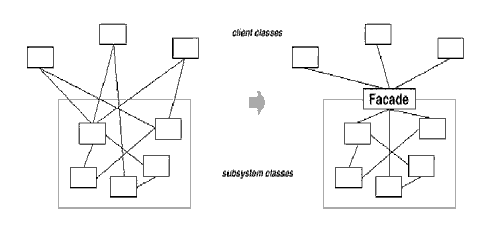public class DBCompare { 　　Connection conn = null; 　　PreparedStatement prep = null; 　　ResultSet rset = null; 　　try { 　　 　　Class.forName( "" ).newInstance(); 　　　　 conn = DriverManager.getConnection( "" ); 　　　　 　　　　 String sql = "SELECT * FROM WHERE = ?"; 　　　　 prep = conn.prepareStatement( sql ); 　　　　 prep.setString( 1, "" ); 　　　　 rset = prep.executeQuery(); 　　　　 if( rset.next() ) { 　　　　　　　　System.out.println( rset.getString( "

 public class DBCompare { 　 String sql = "SELECT * FROM WHERE = ?";　　 　　try { 　　 　　Mysql msql=new mysql(sql); 　　　　 msql.setString( 1, "" ); 　　　　 rset = msql.executeQuery(); 　　　　 if( rset.next() ) { 　　　　　　　　System.out.println( rset.getString( "